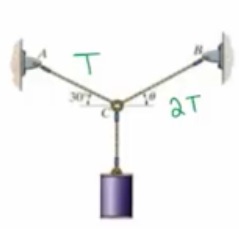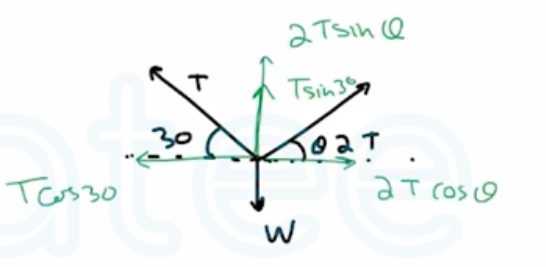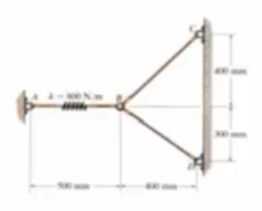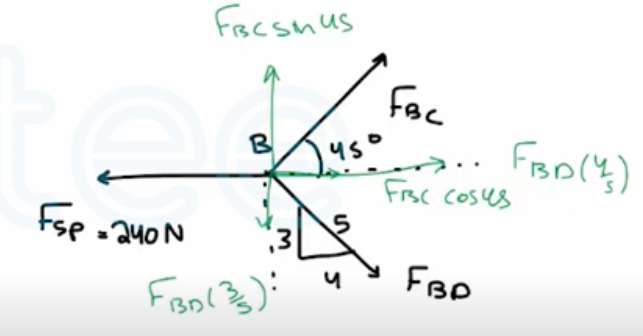Need Help?

Subscribe to Statics

###### \${selected_topic_name}
• Notes

If cable $C B$ is subjected to a tension that is twice that of cable  $C A,$ determine the angle $\theta$ for equilibrium of the 10-kg cylinder. Also, what are the tensions in wires $C A$ and $C B$ ?$\stackrel{+}{\rightarrow}\sum F_{x}=0$

$2Tcos \theta-T cos 30=0$$2 T cos \theta=T cos 30$

$\cos \theta=0.433$

$∴ \theta=\cos ^{-1} 0.433=64.3^{\circ}$

$+\uparrow\sum F_{y}=0 \quad \longrightarrow 2 T sin \theta+T sin 30- W=0$

$2.3T=98.1\longrightarrow T=\frac{98.1}{2.3}=42.6N$

$F_{AC}=T=42.6N$

$F_{C B}=2 T=2 * 42.6=85.2 N$

The spring has a stiffness of  $k=800 \mathrm{N} / \mathrm{m}$ and an unstretched length of  $200 \mathrm{mm}$ . Determine the foree in cables $B C$  and  $B D$ when the spring is held in the position shown.$s=l-l_{0}=0.5-0.2=0.3 \mathrm{m}$

$F_{s p}=k s=800 * 0.3=240 \mathrm{N}$

$\stackrel{+}{\rightarrow}\sum F_{x}=0\longrightarrow F_{BD}\frac{4}{5}+F_{BC} cos 45=0$$0.7071\ F_{BC}+0.8\ F_{BD}=240\longrightarrow$

$+\uparrow\sum F_{y}=0 \rightarrow F_{BC} sin 45-F_{BD}\frac{3}{5}=0$

$F_{B C}=0.8485 \ F_{BD} \longrightarrow$

$∴ \quad F_{B D}=171 \quad N$

$∴ \quad F_{B C}=145 \quad N$